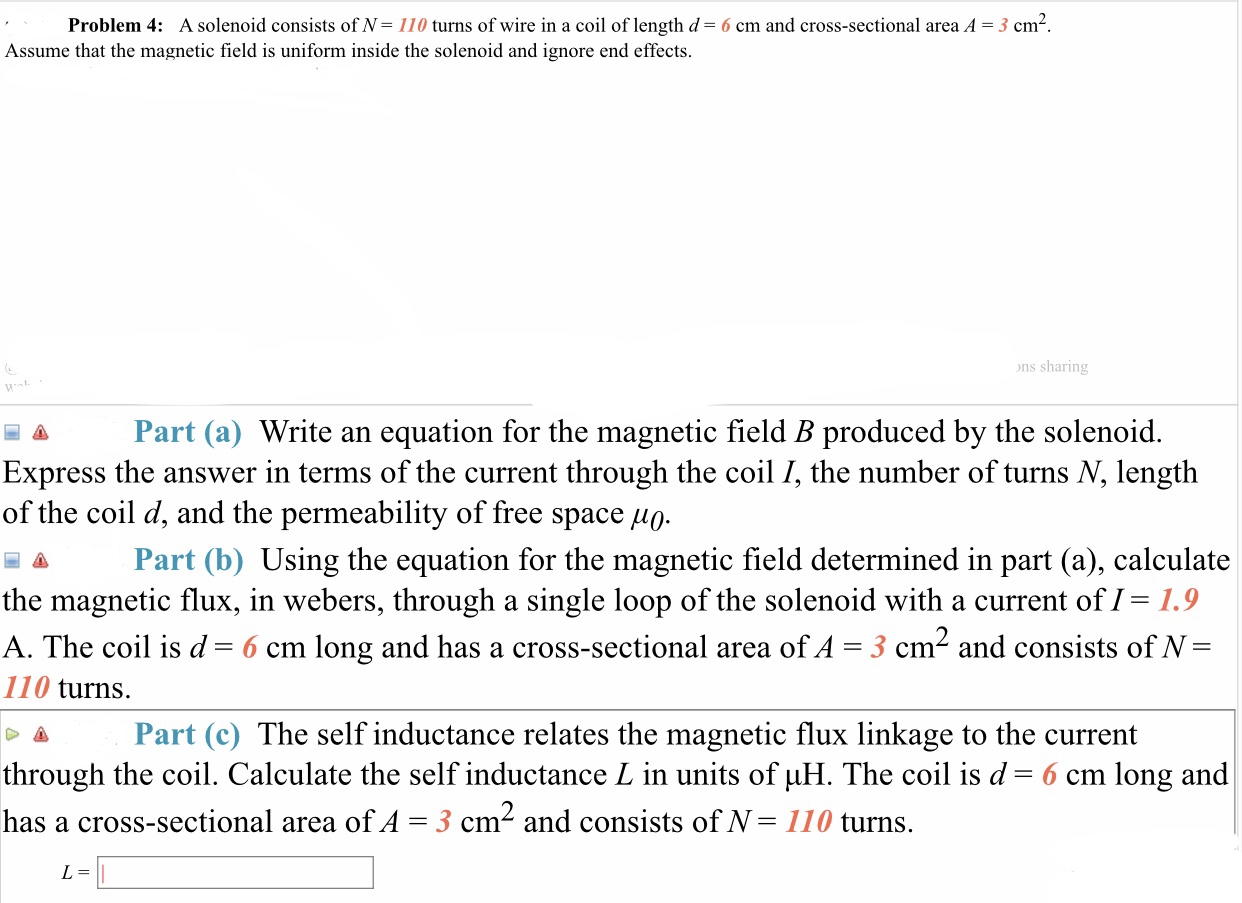# Problem 4: A solenoid consists of N= 110 turns of wire in a coil of length d= 6 cm and cross-sectional area A = 3 cm Assume that the magnetic field is uniform inside the solenoid and ignore end effects ons sharing Part (a) Write an equation for the magnetic field B produced by the solenoid. Express the answer in terms of the current through the coil /, the number of turns N, length of the coil d, and the permeability of free space μ0 a Part (b) Using the equation for the magnetic field determined in part (a), calculate the magnetic flux, in webers, through a single loop of the solenoid with a current of I- 1.9 A. The coil is d= 6 cm long and has a cross-sectional area ofA = 3 cm2 and consists of N 110 turns. Part (c) The self inductance relates the magnetic flux linkage to the current through the coil. Calculate the self inductance L in units of μΗ. The coil is d-6 cm long and has a cross-sectional area of A-3 cm and consists of N- 110 turns.

Questionhelp_outlineImage TranscriptioncloseProblem 4: A solenoid consists of N= 110 turns of wire in a coil of length d= 6 cm and cross-sectional area A = 3 cm Assume that the magnetic field is uniform inside the solenoid and ignore end effects ons sharing Part (a) Write an equation for the magnetic field B produced by the solenoid. Express the answer in terms of the current through the coil /, the number of turns N, length of the coil d, and the permeability of free space μ0 a Part (b) Using the equation for the magnetic field determined in part (a), calculate the magnetic flux, in webers, through a single loop of the solenoid with a current of I- 1.9 A. The coil is d= 6 cm long and has a cross-sectional area ofA = 3 cm2 and consists of N 110 turns. Part (c) The self inductance relates the magnetic flux linkage to the current through the coil. Calculate the self inductance L in units of μΗ. The coil is d-6 cm long and has a cross-sectional area of A-3 cm and consists of N- 110 turns. fullscreen

### Want to see the step-by-step answer?